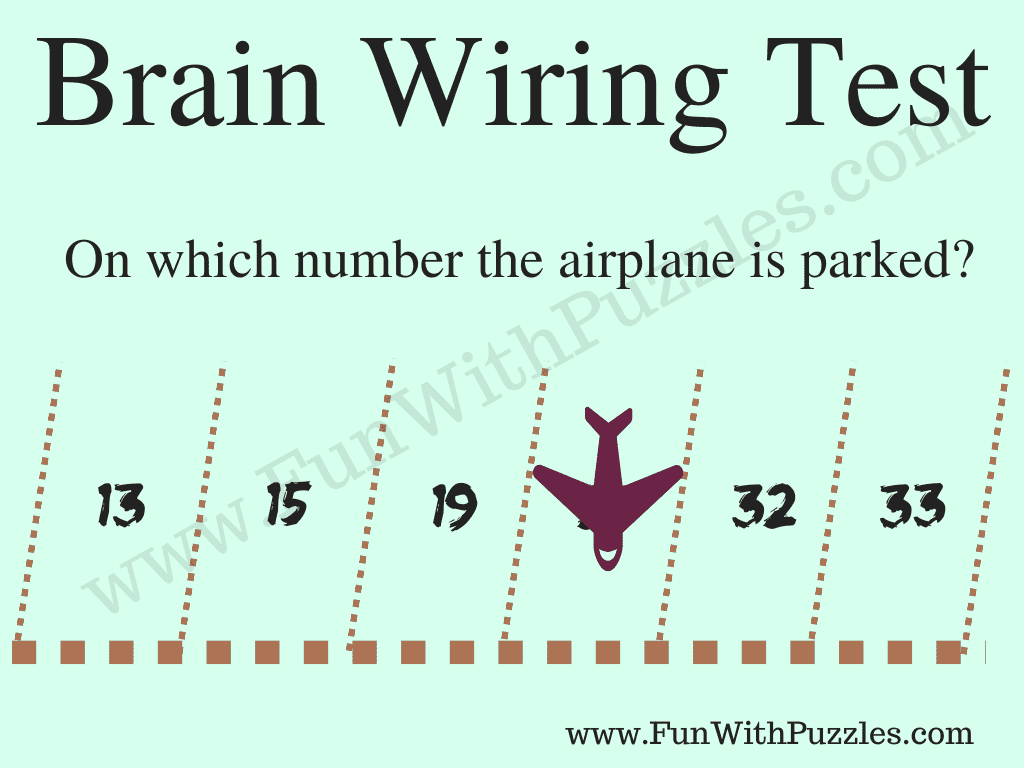Here is the Parking Puzzle of Mathematical to test your brain wiring. This is the parking puzzle in which your need to calculate the parking number on which the airplane is parked. In fact, is the puzzle in a mathematical sequence. In which you need to study the pattern in the given parking slots and then tell the number of the hidden parking slot on which the airplane is parked. Can you solve this number sequence parking puzzle?Can you calculate on which number the airplane is parked?

The answer to this "Brain Wiring Test", can be viewed by clicking on the answer button.